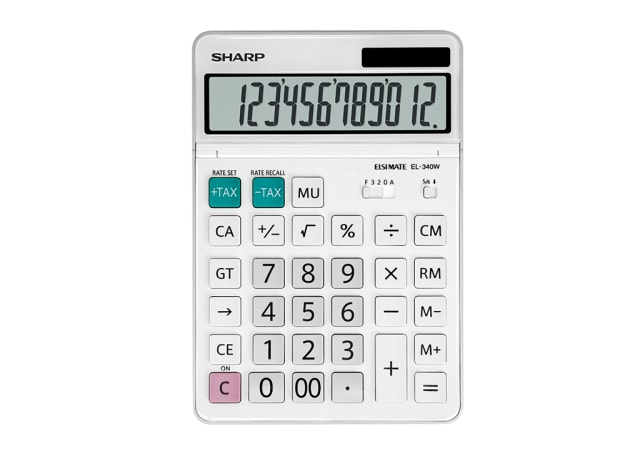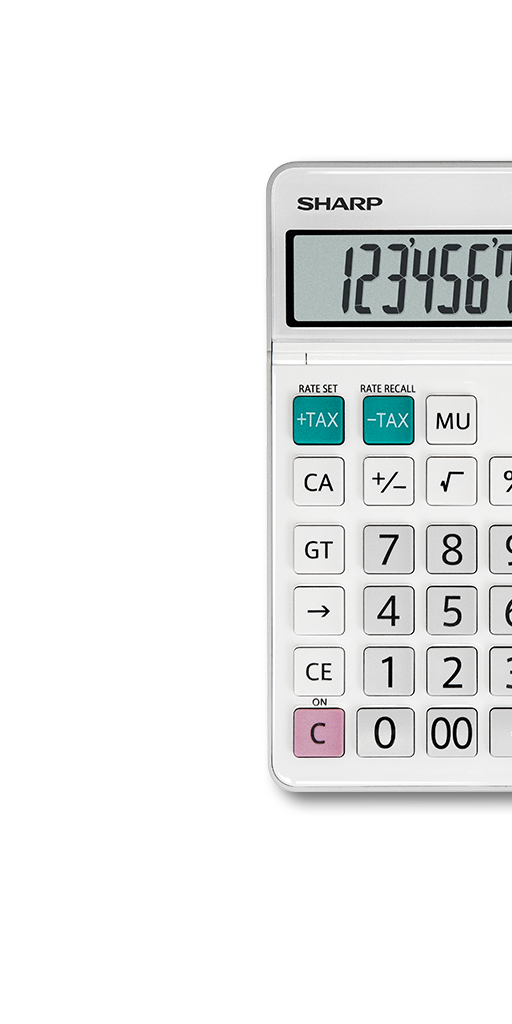EL340WB

New desktop calculator with unique attractive design, basic mathematic and business functions and large tilt adjustable 12-digit display.

POA## LCD Display## Twin Powered## Markup Calculation• Perfect for business professionals at home or in the office, this calculator can be used for calculating tax, margin/ profit, currency conversion etc.
• LCD display showing up to 12 numbers at a time, display can be tilted and adjusted on an angle for comfortable reading.
• Solar powered with 1 x CR2032 battery as backup, calculator can be used both during the day and night. Battery is secured with child-safe lock. Operating time is approximately 5 years with 1 hour of daily use, using the alkaline battery only.
• 4-key memory keeps often-used figures accessible for speedier calculations.
• Adjustable display angle for personal preference.
• Use the decimal settings to select the right option for your accounting tasks. Can be set to floating point, 3/2/0 decimal positions, or adding mode (two decimal places).
• This feature counts the number of items entered. Great for stock counting or accounting purpose.
• Mark up: calculating gross profit, margin gained, or calculating cost. Perfect for using in business
environments.

Grand total: to calculate the cumulative total of your calculations.

E.g. [GT] [GT] [CE] [number] [+] [number] [=] gives you first number
[number] [-] [number] [=] gives you second number
[number] [+] [number] [=] gives you third number
[GT] gives you total of all numbers added together from above

This function is great for when needing to calculate the total profit of a shop subtracting all expenses.

• The square root of a number is a value that when multiplied by itself equals the original number. Great for jobs like architects, carpenters and engineers.
• For easier reading of long, multi-digit numbers, "," or " , " is inserted for each group of three digits. E.g. 10 thousand will displayed as 10,000.
• The calculator powers off automatically after approximately 7 minutes to save energy and prolong battery life.
• To input two zeros by pressing the key once e.g. to input 100,  
• To calculate certain percentage of a number e.g. 100 + 10% gives you 110. Great function for calculating before or after tax amount of a certain item.
Calculator Types
Display
12 Digit Tilt Display
Power Source
Solar / CR2032 x 1
Double Zero Key
Yes
Memory
4
Percentage
Yes
Square Root
Yes
Auto Power Off
Yes
Decimal Setting
Item Count / GT
Yes / Yes
Punctuation
3 Digit